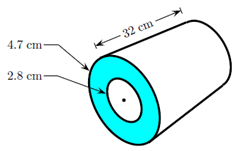# Problem: A piece of pipe has an outer radius of 4.7 cm, an inner radius of 2.8 cm, and length of 32 cm as shown in the figure. What is the mass of this pipe? Assume its density is 7.8 g/cm3.

###### FREE Expert Solution
86% (303 ratings)
###### Problem Details

A piece of pipe has an outer radius of 4.7 cm, an inner radius of 2.8 cm, and length of 32 cm as shown in the figure. What is the mass of this pipe? Assume its density is 7.8 g/cm3.Frequently Asked Questions

What scientific concept do you need to know in order to solve this problem?

Our tutors have indicated that to solve this problem you will need to apply the Density concept. You can view video lessons to learn Density. Or if you need more Density practice, you can also practice Density practice problems.

How long does this problem take to solve?

Our expert Physics tutor, Juan took 3 minutes undefined to solve this problem. You can follow their steps in the video explanation above.

What professor is this problem relevant for?

Based on our data, we think this problem is relevant for Professor Perry's class at TEXAS.